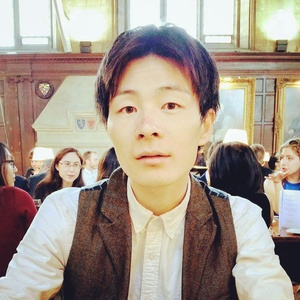Doctoral Student

Student,

Leaving date: 19th December 2018

## Interests

My current research fields are theory (and semantics) of computation, (foundations of) constructive mathematics, (higher) category theory, (categorical) logic and (homotopy) type theory. Specifically, my PhD project is concerned with a mathematical formulation of intensionality and dynamics in logic and computation, in terms of categories in an abstract level and games and strategies in a concrete level (n.b. it is not directly relevant to game theory in economics, but it is based on game semantics for logic and computation). Traditionally, such intensional and dynamic aspects have been studied syntactically, but I aim to capture their essences as mathematical (semantic or syntax-independent) concepts just like any concept in pure mathematics such as differential and integral in calculus and homotopy in topology, ignoring superfluous syntactic formalisms. Also, algebraic and categorical structures that naturally arise thereof are concepts of interest. This article is my first attempt on this research topic; it fomulates step-by-step computational processes in a simple programming language for Heyting arithmetic in a syntax-independent manner.

Moreover, based on the observation that my variant of games and strategies may capture ``atomic steps'' in computation, I have defined an intrinsic (in the sense that it does not have recourse to the standard ``Church-Turing computability'') notion of ``effective computability'' in game semantics. As a consequence, I have formulated a general notion of ``algorithms'' under the name of effective strategies in a non-inductive and syntax-independent manner, giving rise to a mathematical model of computation in the same sense as Turing machines but beyond ``classical computation'', e.g., higher-order one. It subsumes computation of the language PCF, and so it is in particular Turing complete. See this article for this topic.

As another direction, I have developed a generalization of an existing game semantics to interpret intuitionistic type theory (ITT), a well-known foundation of constructive mathematics. This work, incorporating the ``dynamic structure'' of games and strategies described above, would formulate the standard semantics of ITT in terms of a mathematical (semantic) model of computation. See this article for this topic.

## Biography

I earned an undergraduate degree in computer scinece at Hokkaido University, in which I was awarded W. Wheeler Prize and Nitobe Prize, with one-year study in pure mathematics at University of Wisconsin-Madison (GPA 4.00/4.00). During those days, very fortunately, I had exellent mentors, Prof. Thomas Zeugmann and Prof. Ken Ono, who taught me a lot about mathematics and theoretical computer science beyond the curriculum through one-on-one interactions. Also, I wrote a bachelor thesis on computational group theory with Prof. Shin-ichi Minato. I did not earn a master degree and directly came to the PhD in Oxford, for which I have been supported by Funai Overseas Scholarship.